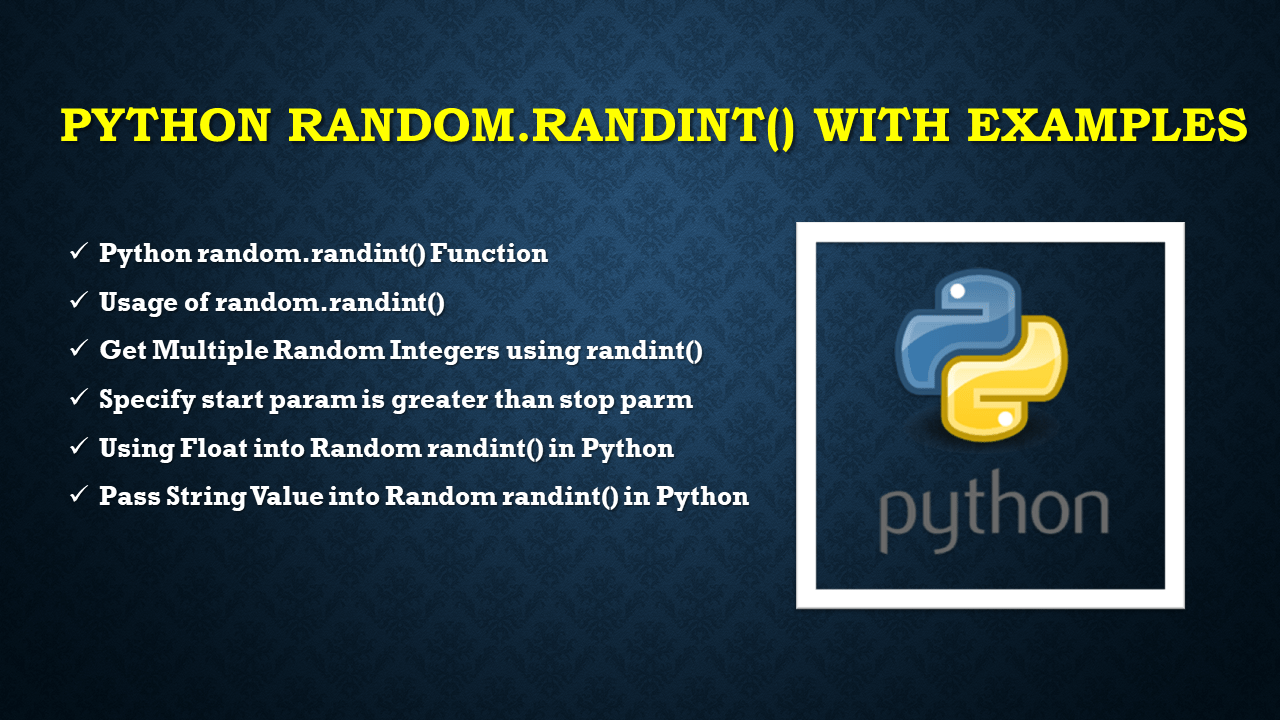# Python random.randint() with Examples

Python `random.randint()` function is available in the random module, which is used to generate the random integer value between the two `start` and `stop` ranges (including both ranges) provided as two parameters. This is one of the most common function to generate a random number between a range of values.

In this article, I will explain Python random.randint() function by using its syntax and parameters and generating the random integer within a given range.

## 1. Quick Examples of random.randint()

Following are the quick examples of random.randint().

``````
# Following are the quick examples of random.randint()
import random

# Example 1: Generate random integer using randint()
random_value= random.randint(20,50)
print(random_value)

# Example 2: Generate multiple Random integers
start = 20
stop = 40
for i in range(5):
print(random.randint(start, stop))
``````

## 2. Python random.randint() Function

The `randint()` from a random module is used to generate the random integer from the given range of integers. It takes start and end numeric values as its parameters, so that a random integer is generated within this specified range.

• `Note1:` If we specify parameters other than the numeric values, `TypeError` will be returned.
• `Note2:` If we specify the float values as its parameters, it will return the `ValueError `.

### 2.1 Syntax of random.randint()

Following the syntax of random.randint().

``````
# Syntax of random.randint()
random.randint(start, end)
``````

### 2.2 Parameters of random.randint()

It takes two parameters.

• `start:` It specifies the starting point of the range and it must be an integer.
• `stop:` It specifies the ending point of the range and it must be an integer.

### 2.3 Return value

It returns random integers from the specified range.

## 3. Usage of random.randint()

Let’s pass a specific range of integers(20, 50) into random.randint(), it will generate a single random integer within a specific range. If you want multiple values, you need to run this statement multiple times.

``````
import random
# Generate random integer using randint()
random_value= random.randint(20,50)
print("Random integer:", random_value)
``````

Yields below output.

## 4. Get Multiple Random Integers using randint()

To get multiple random numbers between two integer values use the randint() function within for loop, each iteration of the loop generates a new random value. Here is an example,

``````
# Import
import random

# Generate multiple random integers
start = 20
stop = 40
for i in range(5):
print(random.randint(start, stop))
``````

Yields below output.

## 5. Specify start param is greater than stop parm

When specifying the range such that the start range is greater than the stop range, this function will give `ValueError`.

``````
# Specify start param greater than stop parm
import random
random_value = random.randint(100,20)
print(random_value)
``````

Here, 100(start) is greater than 20(end). So ValueError: empty range for randrange() (100, 21, -79) is returned.

## 6. Using Float into Random randint() in Python

This function doesn’t take float values as arguments hence when specifying the float type values as a range of values, this function raises an error `ValueError.`

``````
# Using float as arguments randint()
import random
random_value = random.randint(67.20,98.560)
print(random_value)
``````

ValueError: non-integer arg 1 for randrange() is returned.

## 7. Pass String Value into Random randint() in Python

It also doesn’t take the string values, passing string characters as range raises an error. Refer to Random String Generation with Letters and Digits in Python

``````
import random
# Pass string type values into Random randint()
random_value = random.randint(-"A","Z")
print(random_value)
``````

TypeError: bad operand type for unary -: ‘str’ is returned.

## 8. Conclusion

In this article, I have explained random.randint() is used to generate a random integer within the given range of integers. And also explained when we pass parameters other than integers it raises TypeError/ValueError.

Happy Learning!!.

## References